## ML Aggarwal Class 9 Solutions for ICSE Maths Chapter 13 Rectilinear Figures Chapter Test

P.Q.
The interior angles of a polygon add upto 4320°. How many sides does the polygon have ?
Sum of interior angles of a polygon
= (2n – 4) × 90°
⇒ 4320° = (2n – 4) × 90°
⇒ $$\frac{4320^{\circ}}{90^{\circ}}$$ = (2n – 4) ⇒ $$\frac{432}{9}$$ = 2n – 4
⇒ 48 = 2n – 4 ⇒ 48 + 4 = 2n => 52 = 2 n
⇒ 2n = 52 ⇒ n = $$\frac{52}{2}$$ = 26
Hence, the polygon have 26 sides.

P.Q.
If the ratio of an interior angle to the exterior angle of a regular polygon is 5 : 1, find the number of sides.
The ratio of an interior angle to the exterior angle of a regular polygon = 5 : 1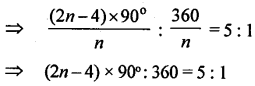Hence, number of sides of regular polygon = 12.

P.Q.
In a pentagon ABCDE, BC || ED and ∠B: ∠A : ∠E = 3 : 4 : 5. Find ∠A.
∵ BC||ED
∴ ∠C +∠D= 180° (Co-interior angles)
But∠A + ∠B + ∠C + ∠D + ∠E = 540°
∴ ∠A + ∠B + ∠E ≠ 180° = 540°
⇒ ∠A + ∠B + ∠E = 540° – 180° = 360°
But ∠B: ∠A = ∠E = 3 : 4 : 5
Let ∠B = 3x, ∠A = 4x and ∠E = 5x∴ 3x + 4x + 5x = 360° ⇒ 12x = 360°
⇒ x = $$\frac{360^{\circ}}{12}$$ = 30°
∴ A = 4x = 4 × 30°= 120°

Question 1.
In the given figure, ABCD is a parallelogram. CB is produced to E such that BE = BC. Prove that AEBD is a parallelogram.Solution:
In the figure, ABCD is a ||gm side CB is produced to E such that BE = BC
BD and AE are joined
To prove: AEBD is a parallelogram
Proof: In ∆AEB and ∆BDC
EB=BC (Given)
∠ABE =∠DCB (Corresponding angles)
AB = DC (Opposite sides of ||gm)
∴ ∆AEB = ∆BDC (SAS axiom)
∴ AE = DB (c.p.c.t.)
But AD = CB = BE (Given)
∵ The opposite sides are equal and ∠AEB = ∠DBC (c.p.c.t.)
But these are corresponding angle
∴ AEBD is a parallelogram

Question 2.
In the given figure, ABC is an isosceles triangle in which AB = AC. AD bisects exterior angle PAC and CD || BA. Show that
(i) ∠DAC = ∠BCA
(ii) ABCD is a parallelogram.
Solution:
Given: In isosceles ∆ABC, AB = AC.
AD is the bisector of ext. ∠PAC and
CD || BA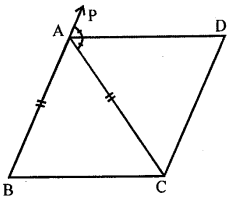To prove: (i) ∠DAC = ∠BCA
(ii) ABCD is a||gm
Proof: In ∆ABC
∵ AB = AC (Given)
∴ ∠C = ∠B (Angles opposite to equal sides)
∵ Ext. ∠PAC = ∠B + ∠C
= ∠C + ∠C = 2∠C = 2∠BCA
∴ 2∠DAC = 2∠BCA
∠DAC = ∠BCA
But these are alternate angles
But AB || AC (Given)
∴ ABCD is a||gm

Question 3.
Prove that the quadrilateral obtained by joining the mid-points of an isosceles trapezium is a rhombus.
Given. ABCD is an isosceles trapezium in which AB || DC and AD = BC
P, Q, R and S are the mid-points of the sides AB, BC, CD and DA respectively PQ, QR, RS and SP are joined.To Prove. PQRS is a rhombus.
Constructions. Join AC and BD.
Proof, ∵ ABCD is an isosceles trapezium
∴ Its diagnoals are equal
∴ AC = BD
Now in ∆ABC,
P and Q are the mid-points of AB and BC
∴ PQ || AC and PQ = $$\frac{1}{2}$$ AC …(i)
S and R mid-points of CD and AD
∴ SR || AC and SR = $$\frac{1}{2}$$ AC …(ii)
from (i) and (ii)
PQ | | SR and PQ = SR
∴ PQRS is a parallelogram
Now in ∆APS and ∆BPQ,
AP = BP (P is mid-point of AB)
AS = BQ Half of equal sides)
∠A = ∠B ( ∵ ABCD is isosceles trapezium)
∴ ∆APS ≅ BPQ
∴ PS = PQ
But there are the adjacent sides of a parallelogram
∴ Sides of PQRS are equal
Hence PQRS is a rhombus.
Hence proved.

Question 4.
Find the size of each lettered angle in the following figures:(i) ∵ CDE is a st. line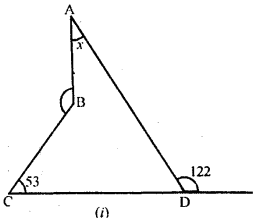∠ABC = 360° – 140° = 220°
(At any point the angle is 360°) …(2)
⇒ 58° + 53° + x + 220° = 36o° [using (1) and (2)]
⇒ 331° + x = 360° ⇒ x = 360° – 331°

(ii) ∵ DE || AB (given)
∴ ∠ECB = ∠CBA (Alternate angles)
⇒ 75° = ∠CBA
∴ ∠CBA =75°
∴ (x + 66°) + (75°) =180° (co-interior angles are supplementary)
⇒ x + 66° + 75° = 180° ⇒ x + 141° = 180°
⇒ x = 180°- 141°
A = 39° …(1)
Now, in ∆AMB,
x + 30° + ∠AMB = 180° (sum of all angles in a triangle is 180°)
⇒ 39° + 30° + ∠AMB = 180° [From (1)]
⇒ 69° + ∠AMB = 180°
⇒ ∠AMB = 180°- 69°
⇒ ∠AMB = 111° ….(2)
∵ ∠AMB = y (vertically opposite angles)
⇒ 111° = y [From (2)]
∴ y = 111°
Hence, A = 39° and y =111°

(iii) In ∆ABD
∠ABD = ∠ADB (∵ equal sides have equal angles opposite to them)
⇒ ∠ABD = 42° [∵ ∠ADB = 42° (given)]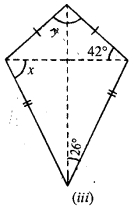(Sum of all angles in a triangle is 180°)
⇒ 42° + 42° + y = 180° ⇒ 84° + y = 180°
⇒ y = 180° – 84° ⇒ y = 96°
∠BCD = 2 × 26° = 52°
In ∠BCD
∵ BC = CD (given)
∴ ∠CBD = ∠CDB = x [equal side have equal angles opposite to them]
∴ ∠CBD + ∠CDB + ∠BCD = 180°
⇒ x + x + 52° = 180° ⇒ 2A = 180° – 52°
⇒ 2x = 128° ⇒ x = $$\frac{128^{\circ}}{2}$$ ⇒ x = 64°
Hence, x = 64° and y = 90°

Question 5.
Find the size of each lettered angle in the following figures :(i) Here AB || CD and BC II AD (given)
∴ ABCD is a || gm
∴ y = 2 × ∠ABD
⇒ y = 2 × 53° = 106° ….(1)
Also, y + ∠DAB = 180°
⇒ 106° + ∠DAB = 180°
⇒ ∠DAB = 180° – 106° ⇒ ∠DAB = 74°
∴ x = $$\frac{1}{2}$$ ∠DAB (∵ AC bisect ∠DAB)⇒ x = $$\frac{1}{2}$$ × 74° = 37°
and ∠D AC = x = 37 ….(2)
∴ ∠DAC = z (Alternate angles) ….(3)
From (2) and (3),
z = 37°
Hence,x = 37°, y = 106°, z = 37°

(ii) ∵ ED is a st. line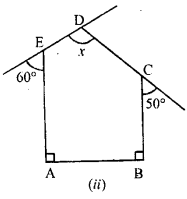∴ 60° + ∠AED = 180° (linear pair)
⇒ ∠AED = 180° – 60°
⇒ ∠AED = 120° …(1)
∵ CD is a st. line
∴ 50° +∠BCD = 180° (linear pair)
⇒ ∠BCD = 180° – 50°
⇒ ∠BCD = 130° ….(2)
In pentagon ABCDE
∠A + ∠B + ∠AED + ∠BCD + x = 540° (Sum of interior angles in pentagon is 540°)
⇒ 90° + 90° + 120° + 130° + x = 540°
⇒ 430° + x = 540° ⇒ x = 540° – 430°
⇒ x = 110°
Hence, value of x = 110°

(iii) In given figure , AD || BC (given)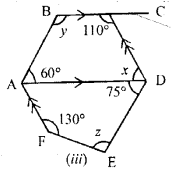∴ 60° + y = 180°and x + 110° = 180°
⇒ y = 180° – 60° and x = 180° – 110°
⇒ y = 120° and x = 70°
∵ CD||AF (given)
∴ ∠FAD = x (Alternate angles)
∠FAD + 75° + z + 130° = 360°
⇒ 70° + 75° + z + 130° = 360° [using(1)]
⇒ 275° + z = 360° ⇒ z = 85°
Hence, x = 10°, y = 120° and z = 85°

Question 6.
In the adjoining figure, ABCD is a rhombus and DCFE is a square. If ∠ABC = 56°, find
(i) ∠DAG
(ii) ∠FEG
(iii) ∠GAC
(iv) ∠AGC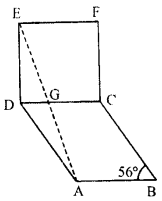Here ABCD and DCFE is a rhombus and square respectively.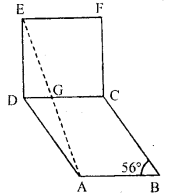∴ AB = BC = DC = AD ….(1)
Also DC = EF = FC = EF ….(2)
From (1) and (2),
AB = BC = DC = AD = EF = FC = EF ….(3)
∠ABC =56° (given)
∠ADC = 56° (opposite angle in rhombus are equal)
∴ ∠EDA = ∠EDC +∠ADC = 90° + 56° = 146°
∠DEA =∠DAE (equal sides have equal opposite angles)
⇒ DEA = DAE = $$\frac{180^{\circ}-\angle \mathrm{EDA}}{2}$$
= $$\frac{180^{\circ}-146^{\circ}}{2}=\frac{34^{\circ}}{2}$$ = 17°
⇒ ∠DAG = 17°
Also, ∠DEG =17°
∴ ∠FEG = ∠E – ∠DEG
= 90°- 17° = 73°
In rhombus ABCD,
∠DAB = 180° – 56° = 124°
∠DAC = $$\frac{124^{\circ}}{2}$$ (∵ AC diagonals bisect the ∠A)
∠DAC =62°
∴ ∠GAC = ∠DAC – ∠DAG
= 62° – 17° = 45°
In ∆EDG,
∠D + ∠DEG + ∠DGE = 180° (Sum of all angles in a triangle is 180°)
⇒ 90° + 17° + ∠DGE = 180°
⇒ ∠DGE = 180° – 107° = 73° ….(4)
Hence, ∠AGC = ∠DGE ….(5) (vertically opposite angles)
From (4) and (5)
∠AGC = 73°

Question 7.
If one angle of a rhombus is 60° and the length of a side is 8 cm, find the/lengths of its diagonals.
Each side of rhombus ABCD is 8 cm. AB = BC = CD = DA = 8cm.Let ∠A = 60°
∴ ∆ABD is an equilateral triangle
∴ AB = BD = AD = 8cm.
∵ Diagonals of a rhombus bisect each other eight angles.
∴ AO = OC, BO = OD=4 cm.
and ∠AOB = 90°
Now in right ∆AOB,
AB = AO2 + OB2 (Pythagoras Theorem)
⇒ (8)2 = AO2 + (4)2
⇒ 64 = AO2 + 16 ,
⇒ AO2 = 64 – 16 = 48 = 16 + 3
∴ AO = $$\sqrt{16 \times 3}=4 \sqrt{3}$$ cm.
But AC = 2 AO
∴ AC = 2 x $$4 \sqrt{3}=8 \sqrt{3}$$ cm

Question 8.
Using ruler and compasses only, construct a parallelogram ABCD with AB = 5 cm, AD = 2.5 cm and ∠BAD = 45°. If the bisector of ∠BAD meets DC at E, prove that ∠AEB is a right angle.
Given : AB = 5 cm, AD = 2.5 cm and ∠BAD =45°.
Required : (i) To construct a parallelogram ABCD.
(ii) If the bisector of ∠BAD meets DC at E then prove that ∠AEB = 90°.Steps of Construction:

• Draw AB = 5.0cm.
• Draw ∠BAP = 45° on side AB.
• Take A as centre and radius 2.5 cm cut the line AP at D.
• Take D as centre and radius 5.0 cm draw an arc.
• Take B as centre and radius equal to 2.5 cm cut the arc of step (4) at C.
• Join BC, and CD.
• ABCD is the required parallelogram.
• Draw the bisector of ∠BAD, which cuts the DC at E.
• Join EB.
• Measure the ∠AEB which is equal to 90°. (Q.E.D.)